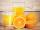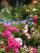# The farmer

The farmer had 140 sheep. For the next year, she decided to change the number of sheep in ratio 10: 7. How many sheep will he have then?

Result

n =  98

#### Solution:

140 = 10/7 * n

10n = 980

n = 98

Calculated by our simple equation calculator.

Leave us a comment of example and its solution (i.e. if it is still somewhat unclear...):

Showing 0 comments:Be the first to comment!#### To solve this example are needed these knowledge from mathematics:

Need help calculate sum, simplify or multiply fractions? Try our fraction calculator. Do you have a linear equation or system of equations and looking for its solution? Or do you have quadratic equation?

## Next similar examples:

1. Cleaning windowsCleaning company has to wash all the windows of the school. The first day washes one-sixth of the windows of the school, the next day three more windows than the first day and the remaining 18 windows washes on the third day. Calculate how many windows ha
2. TogetherGrandfather, father, and son are carpenters. They decided to make a wardrobe. Father produces it for 12 hours, his son for 15 hours, and grandfather for 10 hours. How long will they make it together?
3. EquationSolve the equation: 1/2-2/8 = 1/10; Write the result as a decimal number.
4. Unknown number 24f we add 20, we get 50% of its triple. What is this unknown number?
5. FractionsThree-quarters of an unknown number are 4/5. What is 5/6 of this unknown number?
6. UN 1If we add to an unknown number his quarter, we get 210. Identify unknown number.
7. Unknown numberI think the number - its sixth is 3 smaller than its third.
8. Unknown numberIdentify unknown number which 1/5 is 40 greater than one tenth of that number.
9. 1.5 divided1.5 divided by 1 = w divided by 4
10. PearsThere were pears in the basket, I took two-fifths of them, and left six in the basket. How many pears did I take?
11. TripOn the trip drank 3/10 of pupils tea, 2/5 cola, 1/4 mineral water and remaining 3 juice. How many students were on the trip?
12. RosesOn the large rosary was a third white, half red, yellow quarter and six pink. How many roses was in the rosary?
13. Equation with fractionsSolve equation: ? It is equation with fractions.
14. Equation with xSolve the following equation: 2x- (8x + 1) - (x + 2) / 5 = 9
15. Simple equationSolve for x: 3(x + 2) = x - 18
16. EqnSolve equation with fractions: 2x/3-50=40+x/4
17. Simple equation 6Solve equation with one variable: X/2+X/3+X/4=X+4